# Kerala Syllabus 8th Standard Maths Solutions Chapter 3 Polygons

## Kerala State Syllabus 8th Standard Maths Solutions Chapter 3 Polygons

### Polygons Text Book Questions and Answers

Textbook Page No. 49

Class 8 Maths Polygon Kerala Syllabus Question 1.
Find the sum of angles of a polygon with 52 sides?
Solution:
Sum of angles = (52 – 2) 180
= 50 × 180
= 9000

Hsslive Guru 8th Class Maths Kerala Syllabus Question 2.
The sum of angles of a polygon is 8100°. Find the number of its sides?
Solution:
Let sides = n
∴ Sum of angles = 8100
(n – 2) 180 = 8100
n – 2 = $$\frac{8100}{180}$$
= 45
n = 47, Number sides of the polygon = 47

Hss Live Maths 8th Standard Kerala Syllabus Question 3.
Can 1600 be the sum of angles of a polygon. Can 900° be the sum?
Solution:
1600 is not the multiple of 180°. So it cannot be the sum of the angles. 900° is the multiple of 180. So 900° can be the sum of angles of a polygon.

Polygons Chapter For Class 8 Kerala Syllabus  Question 4.
All the angles of a polygon with 20 sides are equal. Find the measure of each angle?
Solution:
Sum of angles of a polygon with 20 sides. = (20 – 2) × 180
= 18 × 180
Each angle = $$\frac{18 \times 180}{20}$$ = 162°

Hss Live Guru 8th Maths Kerala Syllabus Question 5.
The sum of angles in a polygon is 1980. Find the sum of angles of the polygon with one side more. Find the sum with one side less?
Solution:
When one side of a polygon increases, the sum of angle increases by 180°.
∴ Sum of angles = 1980 + 180 = 2160°
When the number of sides decreases by 1, the sum of angles also decreases by 180°.
∴ Sum of angles = 1980 – 180 = 1800°

Textbook Page No. 51

Polygon Chapter Class 8 Kerala Syllabus Question 1.
Two angles of a triangle are 40°, 60° each. Find the measure of the exterior angles?
Solution:
Third angle = 180 – (40 + 60) = 80°
Exterior angles = 180 – 40, 180 – 60,
180 – 80, 140,
ie, 140°, 120° and 100°

Class 8 Polygon Chapter Kerala Syllabus Question 2.
Find all the angles in the figure.Solution:
∠ABC = 180 – 105 = 75°
∠C = 180 – (35 + 75)
= 180° – 110° = 70°
Exterior angle at A = 180° – 35° = 145°
Exterior angle at B = 180° – 75° = 105°
Exterior angle at C = 180° – 70°
= 110°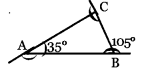Hsslive Maths Class 8 Kerala Syllabus Question 3.
Find all the exterior angles of the quadrilateral in the figure.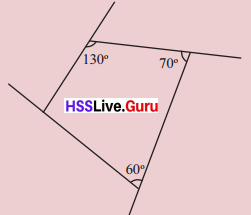Solution:
4th angle = 360 – (130 + 70 + 60) = 100°
Exterior angles = 120°, 110°, 50°, 8o°

8th Class Maths Notes Kerala Syllabus Question 4.
Find all the angles of the given diagrams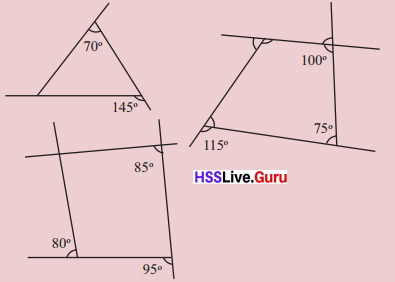Solution:
(i)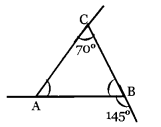Angles of the triangle are 70°, 35°, 75°
Exterior angles are 35°, 110°, 105°

(ii)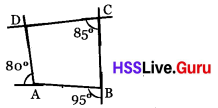∠C = 85°, ∠B = 85°, ∠A = 100°, ∠D = 90°
Exterior angles are 95°, 80°, 90°, 95°
All angles at D are 90°
Angles at C are 85°, 95°, 85°, 95°

(iii)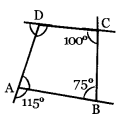Angles of the quadrilateral are :
∠ A = 65°, ∠B = 75s°, ∠C = 100°, ∠D = 120°
Exterior angles are 115°, 105°, 80°, 60°
Angles at C are 100°, 80°, 100°, 80°

Hss Live Guru 8 Maths Kerala Syllabus  Question 5.
Prove that in a triangle, the exterior angle at a vertex is the sum of interior angles at the other two vertices
Solution: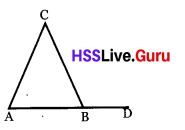∠DBC + ∠ABC = 180° (linear pair)
∠A + ∠C + ∠ABC = 180° (Angles of a triangle)
∴ ∠A + ∠C = ∠DBC
So in a triangle the exterior angle at a vertex is the sum of interior angles at the other two vertices.

Textbook Page No. 54

Hsslive Guru 8th Maths Kerala Syllabus Question 1.
All the angles of an eighteen sided polygon are equal. Find each exterior and interior angles?
Solution:
The angles of the polygon are equal. So the exterior angles are also equal. Sum of the exterior angles = 360°
Measurement of an interior angle = $$\frac{360}{18}$$ = 20°

Hsslive Guru 8 Maths Kerala Syllabus Question 2.
The sides PQ and RS of the quadrilateral PQRS are parallel. Find all the exterior and interior angles of the quadrilateral.
Solution: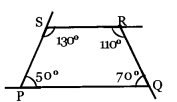PQ and RS are parallel to each other ∠P = 500, The exterior angle at S = 500
∴ ∠S = 130°
∠Q = 360 – (50 + 130 + 110)
= 360 – 290 = 70°
The angles of the quadilateral are ∠P = 50°, ∠Q = 70°, ∠R = 110°, and ∠S = 130°
The exterior angles are 130°, 110°, 70°, 50°

Hss Live Guru Class 8 Maths Kerala Syllabus Question 3.Draw a quadrilateral and mark the exterior angles at two vertices. Is there any relationship between the sum of these angles and the sum of the interior angles at the other two vertices?
Solution:Sum of the angles of the quadrilateral = 360°
Sum of the exterior angles = 360°
Let the sum of two exterior angles = x
The sum of two interior angles at the same vertex = 360 – x. So the sum of the other two interior angles = x. So sum of two exterior angles at two vertices is equal to the sum of two interior angles at the other two vertices.

Kerala Syllabus 8th Standard Maths Notes Question 4.
An exterior angle in a polygon with all angles are equal is twice of an interior angle.
(i) Find the measure of each angle in it?
(ii) Find the number of sides?
Solution:
The exterior angles are equal as all the angles are equal.
Let the interior angle is x, then the exterior angle is 2x.
x + 2x = 3x = 180
∴ x = 60
(i) Interior angles are 6o° each and exterior angles are 120° each.
(ii) The polygon has 3 sides. It is a triangle.

Hsslive Guru Maths 8th Standard Kerala Syllabus Question 5.
The sum of exterior angles in a polygon is twice the sum of the interior angles.
(i) Find how many sides the polygon has?
(ii) Find the number of sides, if the sum of the exterior angles is half of the sum of the interior angles.
(iii) Find the number of sides if the sum of the exterior angles is equal to the sum of the interior angles?
Solution:
The sum of exterior angles in a polygon is 360°.
(i) The sum of the interior angles in a triangle is 180°. It is a triangle and has 3 sides.
(ii) If the sum of the interior angles is 720°, the polygon has six sides.
(iii) If the sum of the interior angles is 360°, the polygon is a quadrilateral it has 4 sides.

Textbook Page No. 58

Hss Live Guru Maths 8th Kerala Syllabus Question 1.
Draw a hexagon with all sides equal but angles different?
Solution:
The sum of angles of a hexagon is 720. Make 720, the sum of 6 different angles. Draw a line with a definite length and make an angle at its end. Then draw the next line and angle. Draw 6 lines continuously and you get a hexagon.

Std 8 Maths Kerala Syllabus Kerala Syllabus Question 2.
Draw a hexagon with all the angles are equal and sides are different.
Solution:
Draw a line. Draw another line by taking an angle 1200 at its one end. Then draw different lines by taking angles 1200. By joining we get a hexagon.

Hsslive 8th Class Maths Kerala Syllabus Question 3.
Find the measurements of each angle in an equal polygon with 15 sides. Find each exterior angles?
Solution:
The sum of angles of a polygon with
15 sides = (15 – 2) × 180
= 13 × 180 = 2340
One angle = $$\frac{2340}{15}$$ = 156°
Exterior angle = 180 – 156 = 24°

Kerala Syllabus 8th Standard Maths Notes Pdf Question 4.
One angle of a regular polygon is 1680. Find the number of its sides?
Solution:
Exterior angle = 180 – 168 = 12°
Sum of exterior angles = 360°
∴ Number of sides = $$\frac{360}{12}$$ = 30

Hsslive Class 8 Maths Kerala Syllabus Question 5.
Can you draw a regular polygon with each exterior angle 6°. Can you draw it if the exterior angle is 7? .
Solution:
Sum of exterior angles = 360°
One of the exterior angle = 6°
Number of sides = $$\frac{360}{6}$$ = 60, yes we can draw; If one exterior angle is 7°
Number of sides = $$\frac{360}{7}$$ = 51.42
Not a whole number. The polygon cannot be drawn.

Question 6.
A regular pentagon and regular hexagon are jointly drawn in the figure. Find PQR?Solution:
Sum of angles of a regular pentagon = (5 – 2) 180°
= 3 × 180 = 540°
One angle of a regular pentagon
= $$\frac{540}{5}$$ = 108°
Sum of angles of a regular hexagon = (6 – 2) 180°
= 4 × 180 = 720°
One of its angle = $$\frac{720}{6}$$ = 120°
∴ ∠PQR = 360 – (108 + 120)
= 360 – 228 = 132°

Question 7.
A square, a regular pentagon and a regular hexagon are jointly drawn in the figure Find ∠BACSolution:
One angle of a regular pentagon = 108°
One angle of a regular hexagon = 120°
One angle of a square = 90°
∴ ∠BAC = 360 – (108 + 20 + 90)
360 – 318 = 42°.

Question 8.
In the figure below, ABCDEF is a regular hexagon. Prove that ∆ BDF is an equilateral triangle?Solution:
Sum of angles of a regular hexagon = (6 – 2) 180 = 720°
= 4 × 180 = 720°
One angle = $$\frac{720}{6}$$ = 120°
consider ∆ EFO
∠E = 120°
∠EFD = ∠EDF = 30°(Angles opposite to equal sides of an isosceles triangle are equal)
Similarly ∠AFB = 30°
∴∠DFB = 120 – (30 + 30) = 60°
∴ ∠FBD = 60°,
∠FDB = 60°
∴ ∆ FDB is an equilateral triangle.

Question 9.
In the figure below ABCDEF is a regular hexagon. Prove that ACDF is rectangle.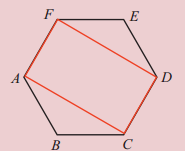Solution:
One angle of a regular hexagon = 120°
∠EFD = ∠EDF = 30°; ∠F = 120°
∴ ∠AFD = 90°
Similarly all the angles of ACDF is 90°
∴ ACDF is a rectangle.

Question 1.
How many triangles can be for made if all the diagonals from a vertex of a 10 sided polygon are drawn?
Solution:
7 diagonals 8 triangles.

Question 2.
The sum of angles of a polygon is x°. Find the sum of angles of the polygon with one side is more. Find the number of sides if one side is less?
Solution:
(x + 180°), (x – 180°)

Question 3.
Can it possible that the external angle of a polygon be 13?
Solution:
$$\frac{360}{13}$$ is a fraction. So it is not possible.

Question 4.
The external angles of a triangle from the three vertices are (2x + 30°), (3x – 10°), 100°. Find the value of x?
Solution:
2x + 30 + 3x – 10 + 100 = 360
5x + 120 = 360
5x = 360 – 120 = 240
∴ x = $$\frac{240}{5}$$ = 48

Question 5.
Draw a circle and construct a regular pentagon with all the vertices in it?
Solution:
Draw circle and construct the pentagon.

Question 6.
Find the sum of angles of a heptagon? Find an angle if all the angles are equal?
Solution:
Sum of angles of a heptagon = (7 – 2) × 180°
= 5 × 180° = 900°
All the angles are equal. So one angle
= $$\frac{900}{7}$$ = 128.571………..

Question 7.
Find the sum of the angles of the polygon with the given sides?
(a) 12
(b) 15
(c) 20
(d) 24
Solution:
(a) Sum of angles = (12 – 2 ) × 180
1800°
(b) Sum of angles = (15 – 2) × 180
= 2340°
(c) Sum of angles = (20 – 2) × 180
= 3240°
(d) Sum of angles = (24 – 2) × 180
= 3960°

Question 8.
The angles of a hexagon are (x – 10)°, x°, (x + 10)°, (x + 20)°, (x + 30)°, and (x + 40)°. Find the value of x?
Solution:
Sum of the angles of a hexagon = (6 – 2) × 180° = 720°
ie, (x – 10) + x + (x + 10) + (x + 20) + (x + 30) + (x + 40) = 720
6x + 90 = 720
ie, 6x = 630; x = 630 ÷ 6
∴ x = 105°

Question 9.
The sum of the angles of a polygon with 12 sides is 1800°. Find the sum of the angles of a polygon with 13 sides?
Solution:
When one side is increased, the angle is increased by 180°.
∴ Sum of the angles of a 13 sides Polygon = 1800° + 180 = 1980°